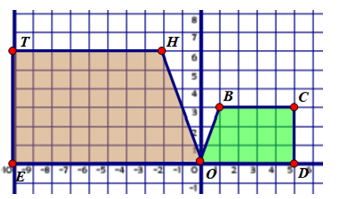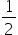Mathematics
Easy

Question

# Quad OBCD is similar to Quad OHTE because:## Quad OBCD can be reflected over the x axis and then rotated 900Quad OBCD can be reflected over the x-axis and then dilated about point O by a scale factor of 2Quad OBCD can be reflected over the y-axis and then dilated about point O by a scale factor of 2Quad OBCD can be reflected over the y-axis and then dilated about point O by a scale factor ofHint:

## The correct answer is: Quad OBCD can be reflected over the y-axis and then dilated about point O by a scale factor of 2

### Related Questions to study#### With Turito Foundation.#### Get an Expert Advice From Turito.